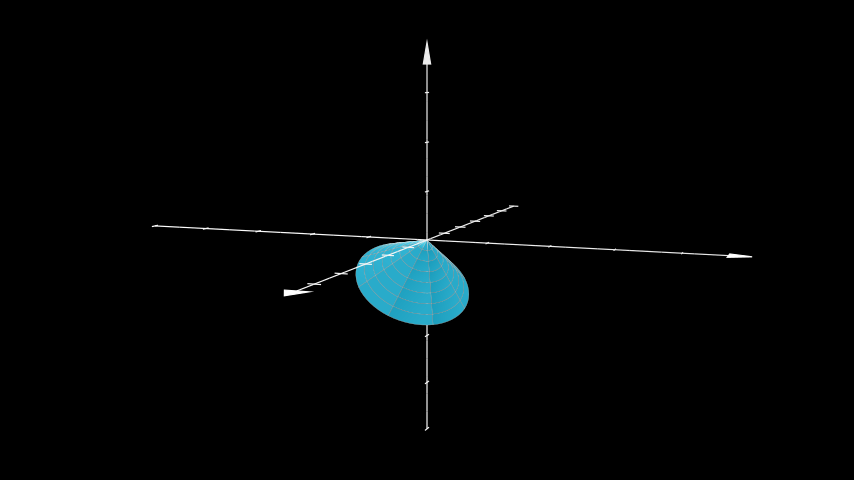# Cone¶

Qualified name: `manim.mobject.three\_dimensions.Cone`

class Cone(base_radius=1, height=1, direction=array([0., 0., 1.]), show_base=False, v_range=[0, 6.283185307179586], u_min=0, checkerboard_colors=False, **kwargs)[source]

A circular cone. Can be defined using 2 parameters: its height, and its base radius. The polar angle, theta, can be calculated using arctan(base_radius / height) The spherical radius, r, is calculated using the pythagorean theorem.

Examples

Example: ExampleCone```from manim import *

class ExampleCone(ThreeDScene):
def construct(self):
axes = ThreeDAxes()
cone = Cone(direction=X_AXIS+Y_AXIS+2*Z_AXIS)
self.set_camera_orientation(phi=5*PI/11, theta=PI/9)
```
Parameters
• base_radius (`float`) – The base radius from which the cone tapers.

• height (`float`) – The height measured from the plane formed by the base_radius to the apex of the cone.

• direction (`numpy.array`) – The direction of the apex.

• show_base (`bool`) – Whether to show the base plane or not.

• v_range (`Sequence[float]`) – The azimuthal angle to start and end at.

• u_min (`float`) – The radius at the apex.

• checkerboard_colors (`bool`) – Show checkerboard grid texture on the cone.

Methods

 `func` Converts from spherical coordinates to cartesian. `get_direction` Uses `shoelace_direction()` to calculate the direction. `set_direction`

Attributes

 `animate` Used to animate the application of a method. `animation_overrides` `depth` The depth of the mobject. `height` The height of the mobject. `width` The width of the mobject.
func(u, v)[source]

Converts from spherical coordinates to cartesian. :param u: The radius. :type u: `float` :param v: The azimuthal angle. :type v: `float`

get_direction()[source]

Uses `shoelace_direction()` to calculate the direction. The direction of points determines in which direction the object is drawn, clockwise or counterclockwise.

Examples

The default direction of a `Circle` is counterclockwise:

```>>> from manim import Circle
>>> Circle().get_direction()
'CCW'
```
Returns

Either `"CW"` or `"CCW"`.

Return type

`str`